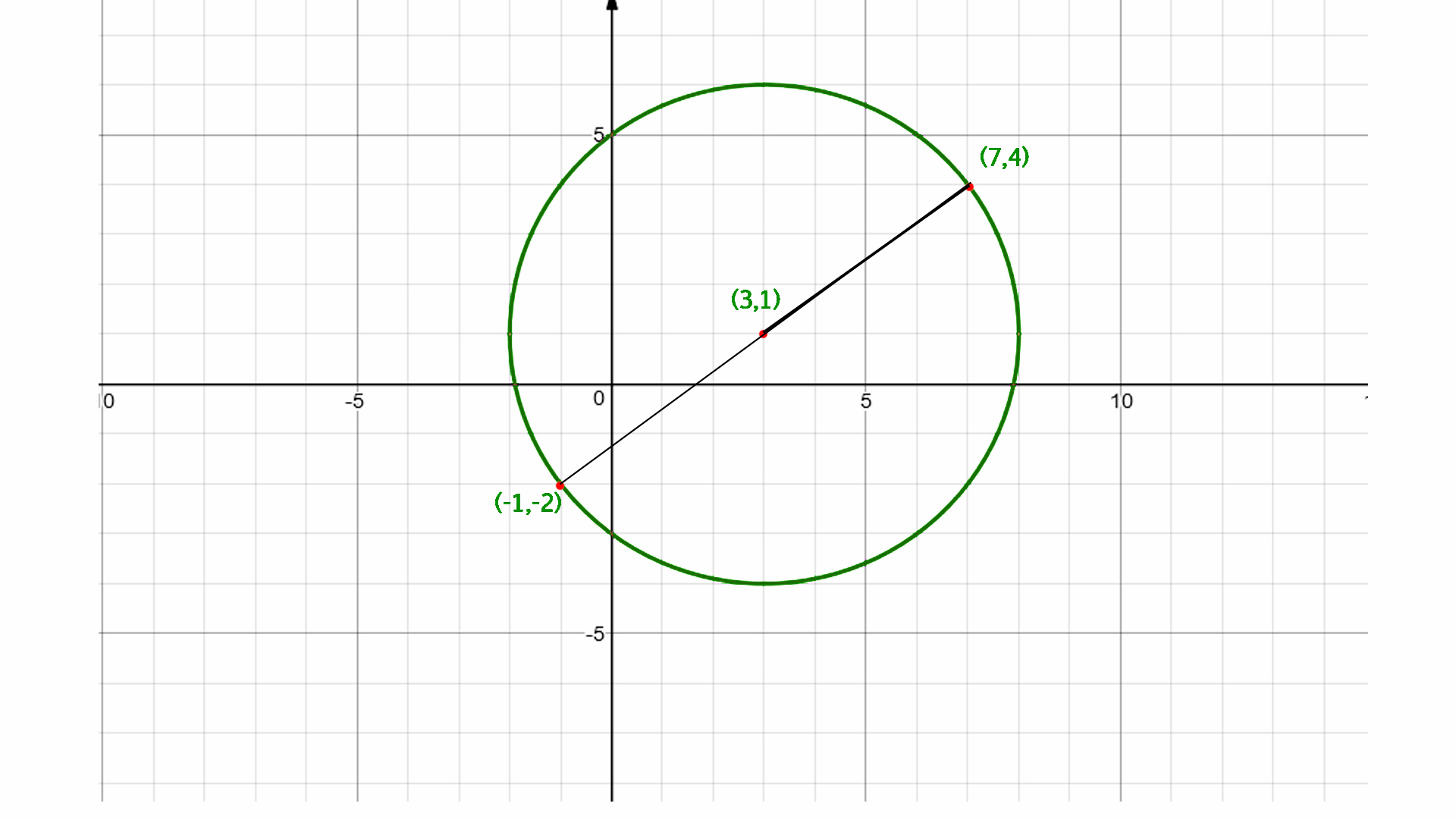# Find the center of the circle using endpoints of diameter

Given two endpoint of diameter of a circle (x1, y1) and (x2, y2) find out the center of a circle.

Examples :

```Input  : x1 = -9, y1 = 3, and
x2 = 5, y2 = –7
Output : -2, –2

Input  :  x1 = 5, y1 = 3 and
x2 = –10 y2 = 4
Output : –2.5, 3.5
```

## Recommended: Please try your approach on {IDE} first, before moving on to the solution.

Midpoint Formula:
The midpoint of two points, (x1, y2) and (x2, y2) is : M = ((x 1 + x 2) / 2, (y 1 + y 2) / 2)

The center of the circle is the mid point of its diameter so we calculate the mid point of its diameter by using midpoint formula.## C++

 `// C++ program to find the ` `// center of the circle ` `#include ` `using` `namespace` `std; ` ` `  `// function to find the ` `// center of the circle ` `void` `center(``int` `x1, ``int` `x2, ` `            ``int` `y1, ``int` `y2) ` `{ ` `     `  `    ``cout << (``float``)(x1 + x2) / 2 <<  ` `          ``", "` `<< (``float``)(y1 + y2) / 2; ` `} ` ` `  `// Driven Program ` `int` `main() ` `{ ` `    ``int` `x1 = -9, y1 = 3, x2 = 5, y2 = -7; ` `    ``center(x1, x2, y1, y2); ` `    ``return` `0; ` `} `

## Java

 `// Java program to find the ` `// center of the circle ` `class` `GFG { ` `     `  `    ``// function to find the ` `    ``// center of the circle ` `    ``static` `void` `center(``int` `x1, ``int` `x2,  ` `                            ``int` `y1, ``int` `y2)  ` `    ``{ ` `         `  `        ``System.out.print((``float``)(x1 + x2) / ``2`  `            ``+ ``", "` `+ (``float``)(y1 + y2) / ``2``); ` `    ``} ` `     `  `    ``// Driver Program to test above function ` `    ``public` `static` `void` `main(String arg[]) { ` `         `  `        ``int` `x1 = -``9``, y1 = ``3``, x2 = ``5``, y2 = -``7``; ` `        ``center(x1, x2, y1, y2); ` `    ``} ` `} ` ` `  `// This code is contributed by Anant Agarwal. `

## Python3

 `# Python3 program to find  ` `# the center of the circle ` ` `  `# Function to find the ` `# center of the circle ` `def` `center(x1, x2, y1, y2) : ` ` `  `    ``print``(``int``((x1 ``+` `x2) ``/` `2``), end``=` `"") ` `    ``print``(``","``, ``int``((y1 ``+` `y2) ``/` `2``) ) ` ` `  `# Driver Code ` `x1 ``=` `-``9``; y1 ``=` `3``; x2 ``=` `5``; y2 ``=` `-``7` `center(x1, x2, y1, y2)  ` ` `  `# This code is contributed by Smitha Dinesh Semwal `

## C#

 `// C# program to find the ` `// center of the circle ` `using` `System; ` ` `  `class` `GFG { ` `     `  `    ``// function to find the ` `    ``// center of the circle ` `    ``static` `void` `center(``int` `x1, ``int` `x2,  ` `                            ``int` `y1, ``int` `y2)  ` `    ``{ ` `         `  `        ``Console.WriteLine((``float``)(x1 + x2) / 2 ` `                ``+ ``", "` `+ (``float``)(y1 + y2) / 2); ` `    ``} ` `     `  `    ``// Driver Program to test above function ` `    ``public` `static` `void` `Main() { ` `         `  `        ``int` `x1 = -9, y1 = 3, x2 = 5, y2 = -7; ` `        ``center(x1, x2, y1, y2); ` `    ``} ` `} ` ` `  `// This code is contributed by vt_m. `

## PHP

 ` `

Output :

```-2, -2
```

My Personal Notes arrow_drop_upCheck out this Author's contributed articles.

If you like GeeksforGeeks and would like to contribute, you can also write an article using contribute.geeksforgeeks.org or mail your article to contribute@geeksforgeeks.org. See your article appearing on the GeeksforGeeks main page and help other Geeks.

Please Improve this article if you find anything incorrect by clicking on the "Improve Article" button below.

Improved By : jit_t

Article Tags :
Practice Tags :

Be the First to upvote.

Please write to us at contribute@geeksforgeeks.org to report any issue with the above content.